# 零基础入门深度学习（四）：卷积神经网络基础之池化和Relu

2020/02/21 16:02下载安装命令

## CPU版本安装命令
pip install -f https://paddlepaddle.org.cn/pip/oschina/cpu paddlepaddle

## GPU版本安装命令
pip install -f https://paddlepaddle.org.cn/pip/oschina/gpu paddlepaddle-gpu

01 导读

1. 深度学习基础知识

2. numpy实现神经网络构建和梯度下降算法

3. 计算机视觉领域主要方向的原理、实践

4. 自然语言处理领域主要方向的原理、实践

5. 个性化推荐算法的原理、实践

02 池化（Pooling）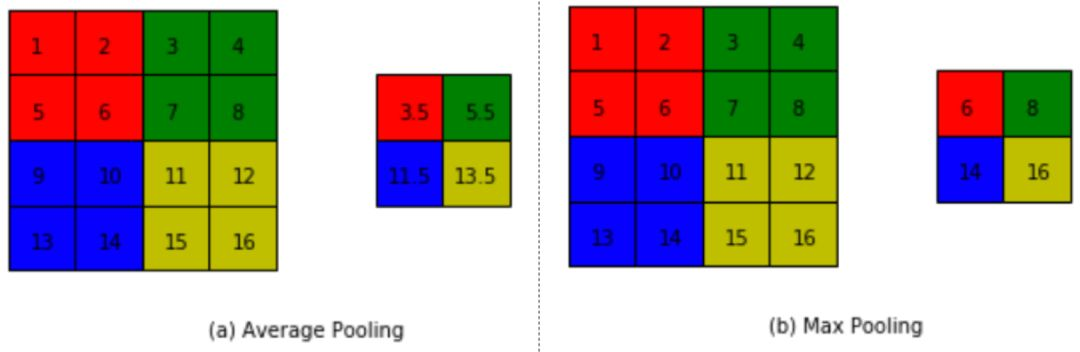• 如图10（a）：平均池化。这里使用大小为的池化窗口，每次移动的步长也为2，对池化窗口内的元素数值取平均，得到相应的输出特征图的像素值。

• 如图10（b）：最大池化。对池化窗口覆盖区域内的元素取最大值，得到输出特征图的像素值。当池化窗口在图片上滑动时，会得到整张输出特征图。池化窗口的大小称为池化大小，用表示。在卷积神经网络中用的比较多的是窗口大小为，步长也为2的池化。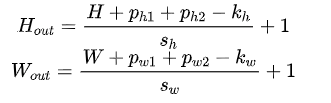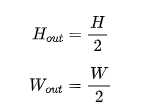03 ReLU激活函数

Sigmoid激活函数定义如下：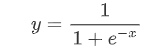ReLU激活函数的定义如下：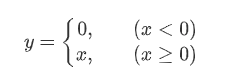# ReLU和Sigmoid激活函数示意图import numpy as npimport matplotlib.pyplot as pltimport matplotlib.patches as patches
plt.figure(figsize=(10, 5))
# 创建数据xx = np.arange(-10, 10, 0.1)
# 计算Sigmoid函数s = 1.0 / (1 + np.exp(0. - x))
# 计算ReLU函数y = np.clip(x, a_min=0., a_max=None)
###################################### 以下部分为画图代码f = plt.subplot(121)plt.plot(x, s, color='r')currentAxis=plt.gca()plt.text(-9.0, 0.9, r'$y=Sigmoid(x)$', fontsize=13)currentAxis.xaxis.set_label_text('x', fontsize=15)currentAxis.yaxis.set_label_text('y', fontsize=15)
f = plt.subplot(122)plt.plot(x, y, color='g')plt.text(-3.0, 9, r'$y=ReLU(x)$', fontsize=13)currentAxis=plt.gca()currentAxis.xaxis.set_label_text('x', fontsize=15)currentAxis.yaxis.set_label_text('y', fontsize=15)
plt.show()


- 梯度消失现象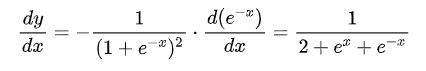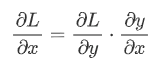ReLU函数则不同，虽然在的地方，ReLU函数的导数为0。但是在的地方，ReLU函数的导数为1，能够将y的梯度完整的传递给x，而不会引起梯度消失。

04 总结

【如何学习】

1. 如何观看配套视频？如何代码实践？

https://aistudio.baidu.com/aistudio/course/introduce/888

1. 学习过程中，有疑问怎么办？

1. 如何学习更多内容？

下载安装命令

## CPU版本安装命令
pip install -f https://paddlepaddle.org.cn/pip/oschina/cpu paddlepaddle

## GPU版本安装命令
pip install -f https://paddlepaddle.org.cn/pip/oschina/gpu paddlepaddle-gpu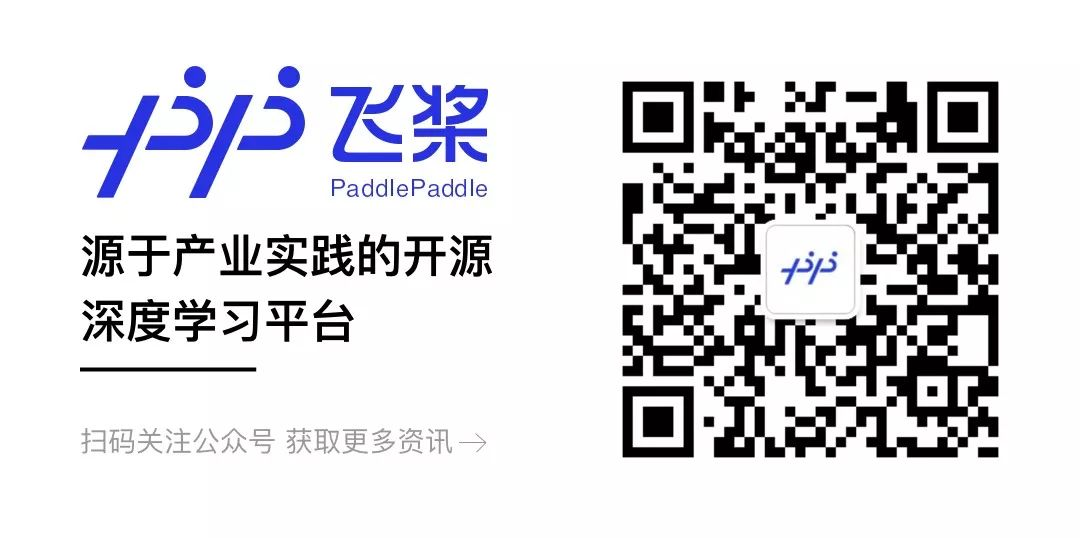>> 访问 PaddlePaddle 官网，了解更多相关内容

0
0 收藏

### 作者的其它热门文章0 评论
0 收藏
0